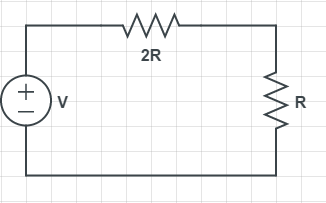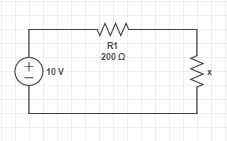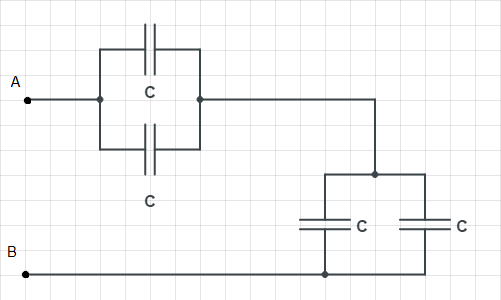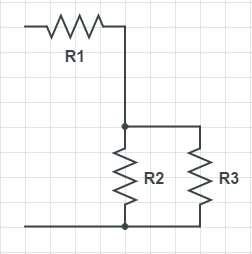# Free SAT II Physics Practice Questions with Solutions DC Electric Circuits

 Sat Physics subject questions on DC electric circuits with detailed solutions, similar to the questions in the SAT test are presented. Answers at the bottom of the page and also detailed solutions and explanations The circuit below is related to questions 1 and 2. V is the e.m.f of the battery, 2R and R are resistors such that the resistance of 2R is double the resistance of R.Fig1. - Electric Circuit Questions 1 and 2. The voltage across resistor R above is given by A) 3V B) V/3 C) V D) V/4 E) V/2 If W is the amount of heat generated during 10 seconds in resistor R included in the circuit in figure 1 above, what would be the amount of heat generated across the same resistor during 20 seconds if V is doubled? A) W B) 2W C) 4W D) 8W E) 80W What is the equivalent resistance between points A and B in the circuit below?A) 0.5 R B) R C) 2 R D) 3 R E) 4 R Find resistance x, in the circuit below, so that the power generated across resistor x is 80% of the total power across resistors R and x?A) 100 Ω B) 200 Ω C) 400 Ω D) 600 Ω E) 800 Ω Two resistors R1 and R2 such that R2 = 3 R1 are in parallel. What is the ratio of the power generated across R1 to the power generated across R2? A) 1 B) 9 C) 3 D) 1 / 3 E) 1 / 9 What is the equivalent capacitance between points A and B?A) C / 2 B) C C) 2 C D) 3 C E) 4 C If 20 Joules of heat is produced across a resistor R during 50 seconds, what amount of heat is produced across the same resistance during 100 seconds if the current across it is doubled? A) 10 J B) 20 J C) 40 J D) 80 J E) 160 J Which of the following is true about electric energy? A) It is stored in resistors. B) It cannot be stored at all. C) It is stored in capacitors. D) It is stored in conducting wires. E) None of the above. Once the switch S1 is closed in the circuit below, which of the following is true about the current through the resistor R1?A) It increases from 0 to V / R1. B) It decreases from V / R1 to 0. C) It stays constant and equal to V / R1. D) It stays constant and equal to (1/2)( V / R1). E) It stays constant and equal to 0. Which of the following is true about the circuit below if R2 > R3?I) The current across R1 is larger than the current across R2 II) The voltage across R3 is equal to the voltage across R2 III) The current across R2 is larger than the current across R3 A) I) and II) only B) II) only C) III) only D) I) only E) I) II) and III) Answers to the Above questions B D C E C B E C B A